# Gravity Forms – How to do advanced number field formula calculations

Gravity Forms provides the ability to perform basic calculations in ‘number’ fields, such as ‘field 1 x 2’ or ‘field 1 + field 2’.

As of Gravity Forms 1.9.15 these formulas do not support any advanced calculations such as ‘if statements’, ‘maximum’ and ‘minimum’.

There is however a way around this that works surprisingly well – by using a combination of multiple ‘number’ fields, each with their own forumlas, and conditional logic you can come up with some pretty advanced calculations.

In this example we will be using calculating half of ‘field 1’ to a maximum of \$2,000.

For example, if ‘field 1’ was \$500 – ‘field 2’ will be \$250. If ‘field 1’ was \$5,000 – ‘field 2’ would be \$2,000 (as it has reached the maximum threshold of \$2,000).

You can see an example of this advanced number field calculation here: http://demo.itsupportguides.com/gravity-forms-infobox-field/single-page-form/

2.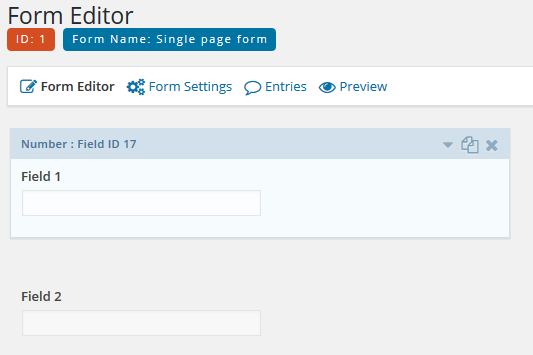3. Calculations can be created on the ‘General’ tab for the number field.
4. Tick the ‘Enable Calculation’ option and enter your formula.You can use the ‘Insert Merge Tag’ drop down to select existing fields in the form. TIP: if you do not see your field, save the form and try again. If you still do not see your field it’s likely that your field is not a supported type – only number, single line text, drop down and radio fields can be used to create formulas.
5. In this example the formula is
6. `{Field 1:17}/2`
7.8. Now on the ‘Advanced’ tab, tick the ‘Enable Conditional Logic’ option – this is where the advanced logic is enter.
9. For this example, because we’re only wanting to use the ‘divided by 2’ formula to a maximum of \$2,000 we will work with the value of \$4,000.
10. Set the ‘All’ drop down to ‘Any’ and enter the following conditions
1. Field 1 less than 4000
2. Field 1 is 4000
3. Field 1 is (empty)
11.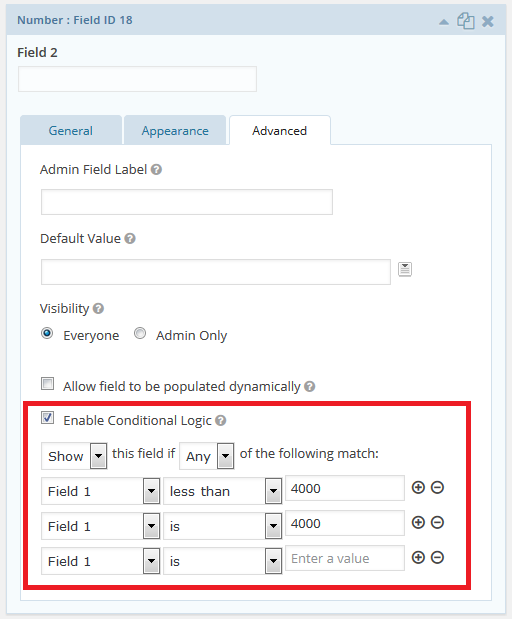12. Now duplicate Field 2 – the new field is where we will handle the calculation if the maximum is more than \$2,000.
13.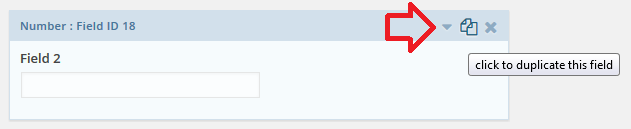14. For this field the calculation formula is simple, because it is a flat amount once it reaches the maximum. Enter
15. `2000`
16.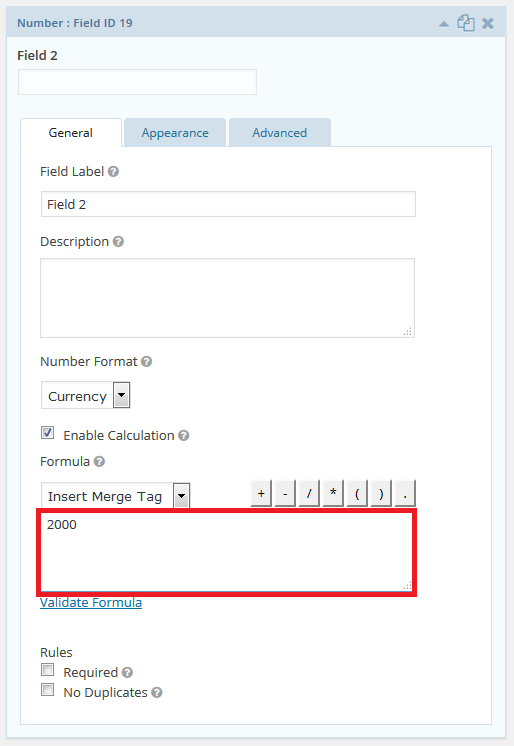17. On the ‘Advanced’ tab remove the existing conditions and replace with ‘if field 1 is greater than 4000’
18.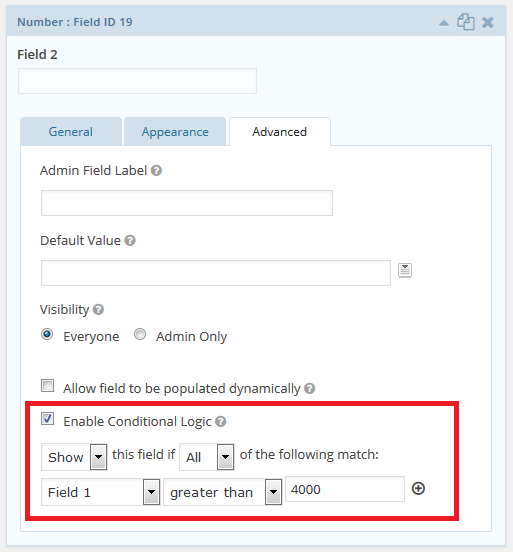19. Save the form and test the results.

You can see an example of this advanced number field calculation here: http://demo.itsupportguides.com/gravity-forms-infobox-field/single-page-form/

## 4 comments on “Gravity Forms – How to do advanced number field formula calculations”

1. Don says:

Can you please help me i am trying to create a formula to subtract a percentage within the Gravity Forms calculation field? I can get an added percentage but not a subtracted percentage

2. streetwise says:

@Walter

Don’t know how you have compared stepFORM with GF, but by the looks of it stepFORM has about 5% of the functionality delivered by GF.

3. Walter says:

It is much easier to edit the formulas for the calculator implemented in stepFORM. It is much easier to configure and there are no special restrictions.

4. Litbea says:

Thanks for the article, it helped me in solving an issue that was driving me nuts for some time!